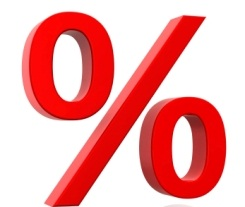# Percent

Percent is used to express ratios in the form of a fraction with 100 as the denominator. In Latin, per cent means per hundred. Percents are usually written down using the internationally recognizable symbol % called the percent sign. Percent express the size of one quantity in relation to another quantity. The same relation can also be expressed with fractions or decimals. Like this:

56% = 0.56 = 56/100

Although percent can range in value from positive to negative infinity (you can actually say that a balloon increased its size by a million percent) and it does not have to be in the form of an integer (there is such a thing as 0.000002 percent), there are situations where the percentage can only be a value between 0 and 100.

When we talk about one part of a larger whole, the whole is considered to be the 100% and the part of that whole cannot be larger than that. For example, if you lose weight, you cannot lose more than 100% of your own weight (in theory – in practice the percent is way smaller than that).

The same thing is true when dealing with probability. The sum of probabilities of all possible outcomes in a single event cannot be greater than 100%. For instance, if you are tossing a coin that can only have two outcomes (heads or tails), there is a 50% chance that the outcome will be heads and a 50% chance the outcome will be tails (sildenafil citrate). If you are throwing a 10-sided dice, each of the possible outcomes has a 10% chance of happening. The sum of all probabilities in an event is always 100%. Never more and never less. And a part of a whole is always smaller than the whole.Percent can also be converted into fractions and/or decimals for easier calculation. If you wish to learn more about that subject, click convert percents, decimals and fractions.

If you wish to practice what you learned about percent, feel free to use the worksheets below.

## Percent exams for teachers

 Worksheet Name File Size Downloads Upload date Percents Percents – easy 0 B 2070 January 1, 1970 Percents – medium 0 B 2289 January 1, 1970 Percents – hard 0 B 1738 January 1, 1970 Fractions, decimals and percents Fractions, decimals and percents – very easy 0 B 1502 January 1, 1970 Fractions, decimals and percents – easy 0 B 1497 January 1, 1970 Fractions, decimals and percents – medium 0 B 1644 January 1, 1970 Fractions, decimals and percents – hard 0 B 1471 January 1, 1970 Fractions, decimals and percents – very_hard 0 B 1543 January 1, 1970

## Percents worksheets for students

 Worksheet Name File Size Downloads Upload date Changing percents to decimals 0 B 2630 January 1, 1970 Changing percents to fractions 0 B 2226 January 1, 1970 Changing decimals to percents 0 B 1792 January 1, 1970 Changing decimals to fractions 0 B 1690 January 1, 1970 Changing fractions to percents 0 B 1493 January 1, 1970 Changing fractions to decimals 0 B 1447 January 1, 1970 Percent problems 0 B 4788 January 1, 1970